NEET  >  Test: Relative Velocity in Two Dimensions (NCERT)

# Test: Relative Velocity in Two Dimensions (NCERT)

Test Description

## 5 Questions MCQ Test | Test: Relative Velocity in Two Dimensions (NCERT)

Test: Relative Velocity in Two Dimensions (NCERT) for NEET 2023 is part of NEET preparation. The Test: Relative Velocity in Two Dimensions (NCERT) questions and answers have been prepared according to the NEET exam syllabus.The Test: Relative Velocity in Two Dimensions (NCERT) MCQs are made for NEET 2023 Exam. Find important definitions, questions, notes, meanings, examples, exercises, MCQs and online tests for Test: Relative Velocity in Two Dimensions (NCERT) below.
Solutions of Test: Relative Velocity in Two Dimensions (NCERT) questions in English are available as part of our course for NEET & Test: Relative Velocity in Two Dimensions (NCERT) solutions in Hindi for NEET course. Download more important topics, notes, lectures and mock test series for NEET Exam by signing up for free. Attempt Test: Relative Velocity in Two Dimensions (NCERT) | 5 questions in 5 minutes | Mock test for NEET preparation | Free important questions MCQ to study for NEET Exam | Download free PDF with solutions
 1 Crore+ students have signed up on EduRev. Have you?
Test: Relative Velocity in Two Dimensions (NCERT) - Question 1

###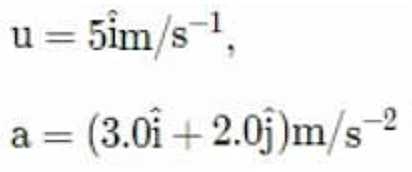The speed of the particle at time= 6 sec

Detailed Solution for Test: Relative Velocity in Two Dimensions (NCERT) - Question 1

Given data,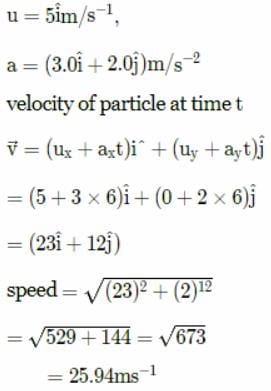Test: Relative Velocity in Two Dimensions (NCERT) - Question 2

### Suppose that two objects A and B are moving with velocities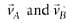(each with respect to some common frame of reference). Let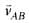represent the velocity of A with respect to B. Then

Detailed Solution for Test: Relative Velocity in Two Dimensions (NCERT) - Question 2

Velocity of object A relative to that of B is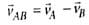Velocity of object B relative to that of A is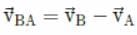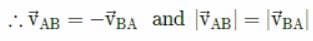Test: Relative Velocity in Two Dimensions (NCERT) - Question 3

### Rain is falling vertically with a speed of 30 ms−1. A woman rides a bicycle with a speed of 12 ms−1 in east to west direction. In which direction she should hold her umbrella?

Detailed Solution for Test: Relative Velocity in Two Dimensions (NCERT) - Question 3

In the figure,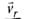represents the velocity of rain and vb​ the velocity of the bicycle, the woman is riding. To protect herself from rain, the woman should hold her umbrella in the direction of the relative velocity of rain with respect to the bicycle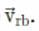From figure,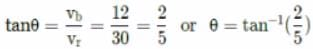Therefore, the woman should hold her umbrella at an angle of tan−1(2/5​)with the vertical towards the west.

Test: Relative Velocity in Two Dimensions (NCERT) - Question 4

A river is flowing from west to east with a speed 5 ms−1. A swimmer can swim in still water at a speed of 10 ms−1. If he wants to start from point A on south bank and reach opposite point B on north bank, in what direction should he swim?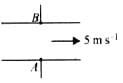Detailed Solution for Test: Relative Velocity in Two Dimensions (NCERT) - Question 4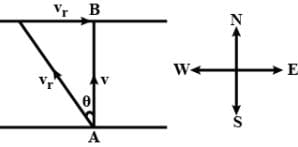Here, Velocity of swimmer in still water, vs​ = 10ms−1
Velocity of water flowing in river, vr ​= 5ms−1
From figure, sinθ = vr/​vs = 5/10 ​= 1/2
θ = sin−1(.1/2​) = 30 west of north

Test: Relative Velocity in Two Dimensions (NCERT) - Question 5

A girl riding a bicycle with a speed of 5 ms−1 towards north direction, observes rain falling vertically down. If she increases her speed to 10 ms−1, rain appears to meet her at 45 to the vertical. What is the speed of the rain?

Detailed Solution for Test: Relative Velocity in Two Dimensions (NCERT) - Question 5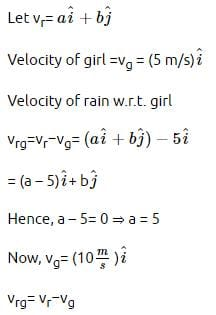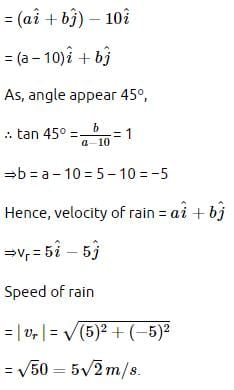Use Code STAYHOME200 and get INR 200 additional OFF Use Coupon Code
Information about Test: Relative Velocity in Two Dimensions (NCERT) Page
In this test you can find the Exam questions for Test: Relative Velocity in Two Dimensions (NCERT) solved & explained in the simplest way possible. Besides giving Questions and answers for Test: Relative Velocity in Two Dimensions (NCERT), EduRev gives you an ample number of Online tests for practice

### How to Prepare for NEET

Read our guide to prepare for NEET which is created by Toppers & the best Teachers